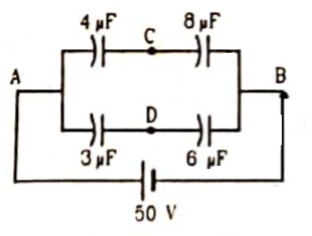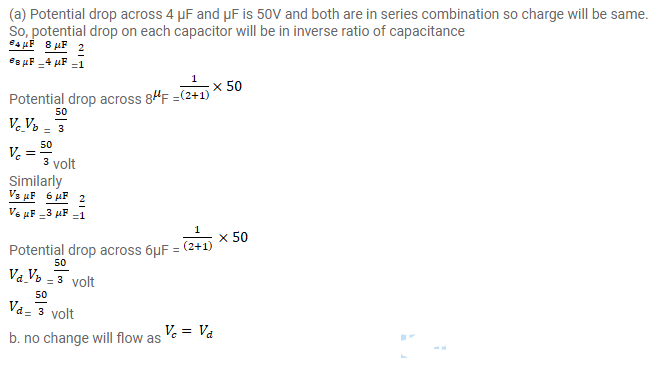# Take the potential of the point B in figure to be zero.Question:

Take the potential of the point B in figure to be zero.

(a) Find the potentials at the points $C$ and D.

(b) If a capacitor is connected between $C$ and $D$, what charge will appear on this capacitor?Solution: